# Chapter 14: ggplot2

Being able to create visualizations (graphical representations) of data is a key step in being able to communicate information and findings to others. In this module you will learn to use the `ggplot2` library to declaratively make beautiful plots or charts of your data. Although R does provide built-in plotting functions, the `ggplot2` library implements the Grammar of Graphics (similar to how `dplyr` implements a Grammar of Data Manipulation; indeed, both packages were developed by the same person). This makes it particularly effective for describing how visualizations should represent data, and has turned it into the preeminent plotting library in R. Learning this library will allow you to easily make nearly any kind of (static) data visualization, customized to your exact specifications.

(Examples in this module adapted from R for Data Science).

## 14.1 A Grammar of Graphics

Just as the grammar of language helps us construct meaningful sentences out of words, the Grammar of Graphics helps us to construct graphical figures out of different visual elements. This grammar gives us a way to talk about parts of a plot: all the circles, lines, arrows, and words that are combined into a diagram for visualizing data. Originally developed by Leland Wilkinson, the Grammar of Graphics was adapted by Hadley Wickham to describe the components of a plot, including

• the data being plotted
• the geometric objects (circles, lines, etc.) that appear on the plot
• a set of mappings from variables in the data to the aesthetics (appearance) of the geometric objects
• a statistical transformation used to calculate the data values used in the plot
• a position adjustment for locating each geometric object on the plot
• a scale (e.g., range of values) for each aesthetic mapping used
• a coordinate system used to organize the geometric objects
• the facets or groups of data shown in different plots

Wickham further organizes these components into layers, where each layer has a single geometric object, statistical transformation, and position adjustment. Following this grammar, you can think of each plot as a set of layers of images, where each image’s appearance is based on some aspect of the data set.

All together, this grammar enables us to discuss what plots look like using a standard set of vocabulary. And like with `dplyr` and the Grammar of Data Manipulation, `ggplot2` uses this grammar directly to declare plots, allowing you to more easily create specific visual images.

## 14.2 Basic Plotting with `ggplot2`

The `ggplot2` library provides a set of declarative functions that mirror the above grammar, enabling us to efficaciously specify what we want a plot to look like (e.g., what data, geometric objects, aesthetics, scales, etc. we want it to have).

`ggplot2` is yet another external package (like `dplyr` and `httr` and `jsonlite`), so you will need to install and load it in order to use it:

``````install.packages("ggplot2")  # once per machine
library("ggplot2")``````

This will make all of the plotting functions you’ll need available.

• Note that the library also comes with a number of built-in data sets. This module will use the provided `mpg` data set as an example, which is a data frame contains information about fuel economy for different cars.

In order to create a plot, you call the `ggplot()` function, specifying the data that you wish to plot. You then add new layers that are geometric objects which will show up on the plot:

``````# plot the `mpg` data set, with highway milage on the x axis and
# engine displacement (power) on the y axis:
ggplot(data = mpg) +
geom_point(mapping = aes(x = displ, y = hwy))``````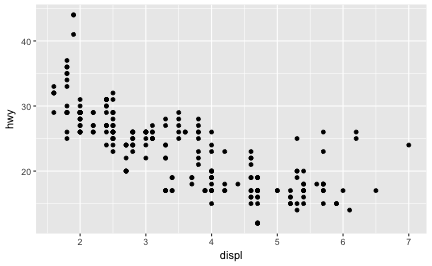plot of chunk basic_mpg

To walk through the above code:

• The `ggplot()` function is passed the data frame to plot as the `data` argument.

• You specify a geometric object (`geom`) by calling one of the many`geom` functions, which are all named `geom_` followed by the name of the kind of geometry you wish to create. For example, `geom_point()` will create a layer with “point” (dot) elements as the geometry. There are large number of these functions; see below for more details.

• For each `geom` you must specify the aesthetic mappings, which is how data from the data frame will be mapped to visual aspects. These mappings are defined using the `aes()` function. The `aes()` function takes a set of arguments (like a list), where the name is the visual property to map to, and the value is the data property to map from.

• Finally, you add `geom` layers to the plot by using the addition (`+`) operator.

Thus basic simple plots can be created simply by specifying a data set, a `geom`, and a set of aesthetic mappings.

• Note that `ggplot2` library does include a `qplot()` function for creating “quick plots”, which acts as a convenient shortcut for making simple, “default”-like plots. However, for this course you should focus on thinking about plots in terms of the Grammar of Graphics and use the `ggplot()` function instead.

### 14.2.1 Aesthetic Mappings

The aesthetic mappings take properties of the data and use them to influence visual channels, such as position, color, size, or shape. Each visual channel can thus encode an aspect of the data and be used to convey information.

All aesthetics for a plot are specified in the `aes()` function call for that `geom` layer. For example, we can add a mapping from the `class` of the cars to the color channel:

``````# color the data by car type
ggplot(data = mpg) +
geom_point(mapping = aes(x = displ, y = hwy, color = class))``````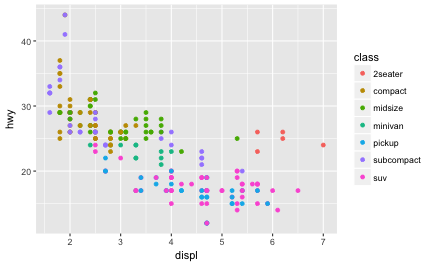plot of chunk aes_color

(`ggplot2` will even create a legend for you!)

Note that using the `aes()` function will cause the visual channel to be based on the data specified in the argument. For example, using `aes(color = "blue")` won’t cause the geometry’s color to be “blue”, but will instead cause the visual channel to be mapped from the vector `c("blue")`—as if we only had a single type of engine that happened to be called “blue”. If you wish to apply an aesthetic property to an entire geometry, you can set that property as an argument to the `geom` method, outside of the `aes()` call:

``````ggplot(data = mpg) +
geom_point(mapping = aes(x = displ, y = hwy), color = "blue")  # blue points!``````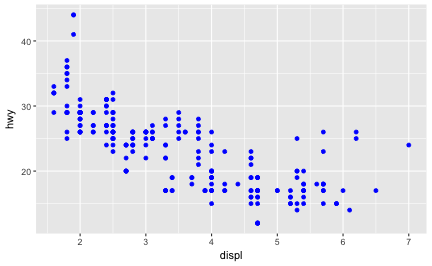plot of chunk color_blue

## 14.3 Complex Plots

Building on these basics, `ggplot2` can be used to build almost any kind of plot you may want. These plots are declared using functions that follow from the Grammar of Graphics.

### 14.3.1 Specifying Geometry

The most obvious distinction between plots is what geometric objects (`geoms`) they include. `ggplot2` supports a number of different types of `geoms`, including:

• `geom_point` for drawing individual points (e.g., a scatter plot)
• `geom_line` for drawing lines (e.g., for a line charts)
• `geom_smooth` for drawing smoothed lines (e.g., for simple trends or approximations)
• `geom_bar` for drawing bars (e.g., for bar charts)
• `geom_polygon` for drawing arbitrary shapes
• `geom_map` for drawing polygons in the shape of a map! (You can access the data to use for these maps by using the `map_data()` function).

Each of these geometries will need to include a set of aesthetic mappings (using the `aes()` function and assigned to the `mapping` argument), though the specific visual properties that the data will map to will vary. For example, you can map data to the `shape` of a `geom_point` (e.g., if they should be circles or squares), or you can map data to the `linetype` of a `geom_line` (e.g., if it is solid or dotted), but not vice versa.

• Almost all `geoms` require an `x` and `y` mapping at the bare minimum.
``````# line chart of milage by engine power
ggplot(data = mpg) +
geom_line(mapping = aes(x = displ, y = hwy))

# bar chart of car type
ggplot(data = mpg) +
geom_bar(mapping = aes(x = class))  # no y mapping needed!``````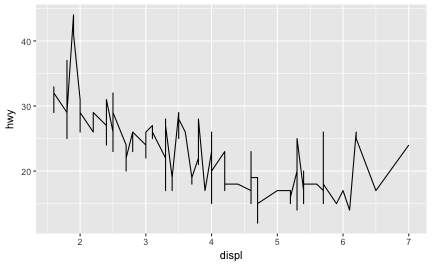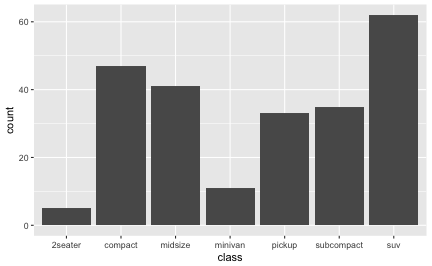What makes this really powerful is that you can add multiple geometries to a plot, thus allowing you to create complex graphics showing multiple aspects of your data

``````#plot with both points and smoothed line
ggplot(data = mpg) +
geom_point(mapping = aes(x = displ, y = hwy)) +
geom_smooth(mapping = aes(x = displ, y = hwy))``````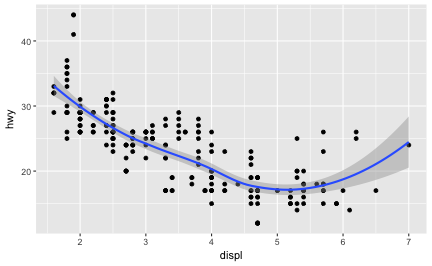plot of chunk multi_geom

Of course the aesthetics for each `geom` can be different, so you could show multiple lines on the same plot (or with different colors, styles, etc). It’s also possible to give each `geom` a different `data` argument, so that you can show multiple data sets in the same plot.

• If you want multiple `geoms` to utilize the same data or aesthetics, you can pass those values as arguments to the `ggplot()` function itself; any `geoms` added to that plot will use the values declared for the whole plot unless overridden by individual specifications.

#### 14.3.1.1 Statistical Transformations

If you look at the above `bar` chart, you’ll notice that the the `y` axis was defined for us as the `count` of elements that have the particular type. This `count` isn’t part of the data set (it’s not a column in `mpg`), but is instead a statistical transformation that the `geom_bar` automatically applies to the data. In particular, it applies the `stat_count` transformation.

`ggplot2` supports many different statistical transformations. For example, the “identity” transformation will leave the data “as is”. You can specify which statistical transformation a `geom` uses by passing it as the `stat` argument:

``````# silly example: bar chart of engine power vs. milage
# (we need the `y` mapping since it is not implied by the stat transform
ggplot(data = mpg) +
geom_bar(mapping = aes(x = displ, y = hwy), stat="identity")``````

Additionally, `ggplot2` contains `stat_` functions (e.g., `stat_identity` for the “identity” transformation) that can be used to specify a layer in the same way a `geom` does:

``````# generate a "binned" (grouped) display of highway milage
ggplot(data = mpg) +
stat_bin(aes(x=hwy, color=hwy), binwidth=4)  # binned into groups of 4 units``````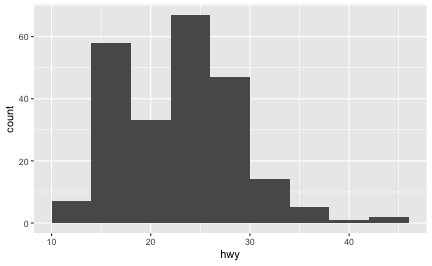plot of chunk stat_summary

Notice the above chart is actually a histogram! Indeed, almost every `stat` transformation corresponds to a particular `geom` (and vice versa) by default. Thus they can often be used interchangeably, depending on how you want to emphasize your layer creation.

``````# these two charts are identical
ggplot(data = mpg) +
geom_bar(mapping = aes(x = class))

ggplot(data = mpg) +
stat_count(mapping = aes(x = class))``````

In addition to a default statistical transformation, each `geom` also has a default position adjustment which specifies a set of “rules” as to how different components should be positioned relative to each other. This position is noticeable in a `geom_bar` if you map a different variable to the color visual channel:

``````# bar chart of milage, colored by engine type
ggplot(data = mpg) +
geom_bar(mapping = aes(x = hwy, fill=class))  # fill color, not outline color``````

The `geom_bar` by default uses a position adjustment of `"stack"`, which makes each rectangle’s height proprotional to its value and stacks them on top of each other. We can use the `position` argument to specify what position adjustment rules to follow: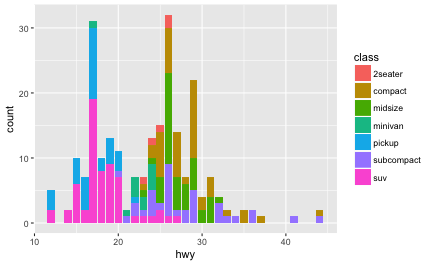``````# a filled bar chart (fill the vertical height)
ggplot(data = mpg) +
geom_bar(mapping = aes(x = hwy, fill=drv), position="fill")

# a dodged bar chart (values next to each other)
# (not great dodging demos in this data set)
ggplot(data = mpg) +
geom_bar(mapping = aes(x = hwy, fill=drv), position="dodge")``````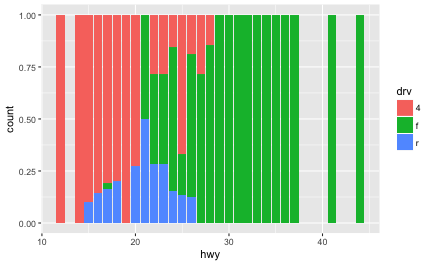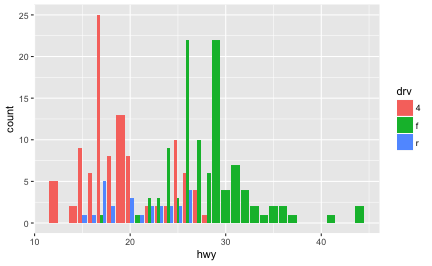Check the documentation for each particular `geom` to learn more about its positioning adjustments.

### 14.3.2 Styling with Scales

Whenever you specify an aesthetic mapping, `ggplot` uses a particular scale to determine the range of values that the data should map to. Thus when you specify

``````# color the data by engine type
ggplot(data = mpg) +
geom_point(mapping = aes(x = displ, y = hwy, color = class))``````

`ggplot` automatically adds a scale for each mapping to the plot:

``````# same as above, with explicit scales
ggplot(data = mpg) +
geom_point(mapping = aes(x = displ, y = hwy, color = class)) +
scale_x_continuous() +
scale_y_continuous() +
scale_colour_discrete()``````

Each scale can be represented by a function with the following name: `scale_`, followed by the name of the aesthetic property, followed by an `_` and the name of the scale. A `continuous` scale will handle things like numeric data (where there is a continuous set of numbers), whereas a `discrete` scale will handle things like colors (since there is a small list of distinct colors).

While the default scales will work fine, it is possible to explicitly add different scales to replace the defaults. For example, you can use a scale to change the direction of an axis:

``````# milage relationship, ordered in reverse
ggplot(data = mpg) +
geom_point(mapping = aes(x = cty, y = hwy)) +
scale_x_reverse()``````

Similarly, you can use `scale_x_log10()` to plot on a logarithmic scale.

You can also use scales to specify the range of values on a axis by passing in a `limits` argument. This is useful for making sure that multiple graphs share scales or formats.

``````# subset data by class
suv = mpg %>% filter(class == "suv")  # suvs
compact = mpg %>% filter(class == "compact")  # compact cars

# scales
x.scale <- scale_x_continuous(limits = range(mpg\$displ))
y.scale <- scale_y_continuous(limits = range(mpg\$hwy))
col.scale <- scale_colour_discrete(limits = unique(mpg\$drv))

ggplot(data = suv) +
geom_point(mapping = aes(x = displ, y = hwy, color = drv)) +
x.scale + y.scale + col.scale

ggplot(data = compact) +
geom_point(mapping = aes(x = displ, y = hwy, color = drv)) +
x.scale + y.scale + col.scale``````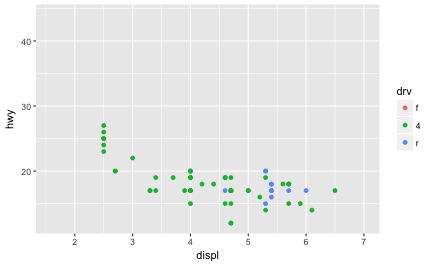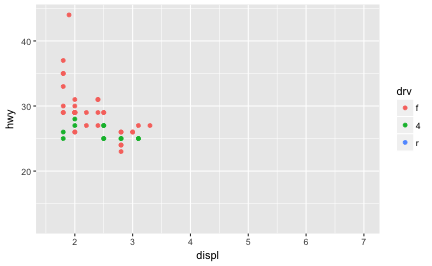Notice how it is easy to compare the two data sets to each other because the axes and colors match!

These scales can also be used to specify the “tick” marks and labels; see the above resources for details. And for further ways specifying where the data appears on the graph, see the “Coordinate Systems” section below.

#### 14.3.2.1 Color Scales

A more common scale to change is which set of colors to use in a plot. While you can use scale functions to specify a list of colors to use, a more common option is to pre-defined palette from colorbrewer.org. These color set have been carefully designed to look good and to be viewable to people with certain forms of color blindness. This color scale is specified with the `scale_color_brewer()` function, passing the `pallete` as an argument.

``````ggplot(data = mpg) +
geom_point(mapping = aes(x = displ, y = hwy, color = class), size=4) +
scale_color_brewer(palette = "Set3")``````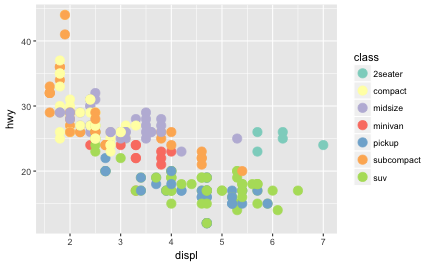plot of chunk brewer_point

Note that you can get the palette name from the colorbrewer website by looking at the `scheme` query parameter in the URL. Or see the diagram here and hover the mouse over each palette for the name.

You can also specify continuous color values by using a gradient scale, or manually specify the colors you want to use as a named vector.

### 14.3.3 Coordinate Systems

The next term from the Grammar of Graphics that can be specified is the coordinate system. As with scales, coordinate systems are specified with functions (that all start with `coord_`) and are added to a `ggplot`. There are a number of different possible coordinate systems to use, including:

• `coord_cartesian` the default cartesian coordinate system, where you specify `x` and `y` values.
• `coord_flip` a cartesian system with the `x` and `y` flipped
• `coord_fixed` a cartesian system with a “fixed” aspect ratio (e.g., 1.78 for a “widescreen” plot)
• `coord_polar` a plot using polar coordinates
• `coord_quickmap` a coordinate system that approximates a good aspect ratio for maps. See documentation for more details.

Most of these system support the `xlim` and `ylim` arguments, which specify the limits for the coordinate system (see above).

### 14.3.4 Facets

Facets are ways of grouping a data plot into multiple different pieces (subplots). This allows you to view a separate plot for each value in a categorical variable. Conceptually, breaking a plot up into facets is similar to using the `group_by()` verb works in `dplyr`, with each facet acting like a level in an R factor.

You can construct a plot with multiple facets by using the `facet_wrap()` function. This will produce a “row” of subplots, one for each categorical variable (the number of rows can be specified with an additional argument):

``````# a plot with facets based on vehicle type.
# similar to what we did with `suv` and `compact`!
ggplot(data = mpg) +
geom_point(mapping = aes(x = displ, y = hwy)) +
facet_wrap(~class)``````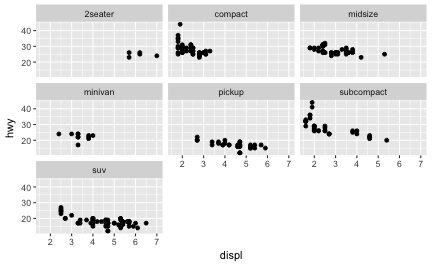plot of chunk facets

Note that the argument to `facet_wrap()` function is written with a tilde (`~`) in front of it. This specifies that the column name should be treated as a formula. A formula is a bit like an “equation” in mathematics; it’s like a string representing what set of operations you want to perform (putting the column name in a string also works in this simple case). Formulas are in fact the same structure used with standard evaluation in `dplyr`; putting a `~` in front of an expression (such as `~ desc(colname)`) allows SE to work.

• tl;dr: put a `~` in front of the column name you want to “group” by.

### 14.3.5 Labels & Annotations

Textual labels and annotations (on the plot, axes, geometry, and legend) are an important part of making a plot understandable and communicating information. Although not an explicit part of the Grammar of Graphics (the would be considered a form of geometry), `ggplot` makes it easy to add such annotations.

You can add titles and axis labels to a chart using the `labs()` function (not `labels`, which is a different R function!):

``````ggplot(data = mpg) +
geom_point(mapping = aes(x = displ, y = hwy, color = class)) +
labs(title = "Fuel Efficiency by Engine Power, 1999-2008",  # plot title
x = "Engine power (litres displacement)",  # x-axis label (with units!)
y = "Fuel Efficiency (miles per gallon)",  # y-axis label (with units!)
color = "Car Type")  # legend label for the "color" property``````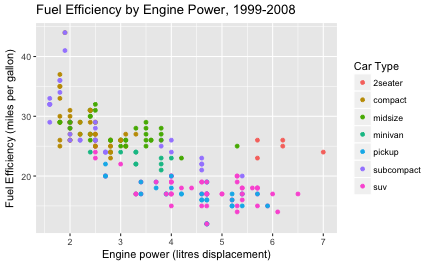plot of chunk labels

It is possible to add labels into the plot itself (e.g., to label each point or line) by adding a new `geom_text` or `geom_label` to the plot; effectively, you’re plotting an extra set of data which happen to be the variable names:

``````# a data table of each car that has best efficiency of its type
best_in_class <- mpg %>%
group_by(class) %>%
filter(row_number(desc(hwy)) == 1)

ggplot(data = mpg, mapping = aes(x = displ, y = hwy)) +  # same mapping for all geoms
geom_point(mapping = aes(color = class)) +
geom_label(data = best_in_class, mapping = aes(label = model), alpha = 0.5)``````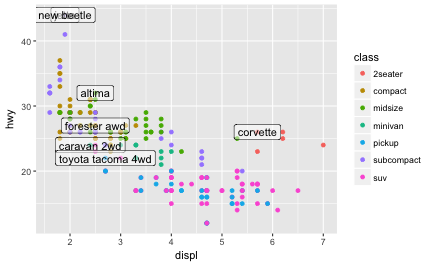plot of chunk annotations

“R for Data Science” (linked in the resources) recommends using the `ggrepel` package to help position labels.

## 14.4 Other Visualization Libraries

`ggplot2` is easily the most popular library for producing data visualizations in R. That said, `ggplot2` is used to produce static visualizations: unchanging “pictures” of plots. Static plots are great for for explanatory visualizations: visualizations that are used to communicate some information—or more commonly, an argument about that information. All of the above visualizations have been ways for us to explain and demonstrate an argument about the data (e.g., the relationship between car engines and fuel efficiency).

Data visualizations can also be highly effective for exploratory analysis, in which the visualization is used as a way to ask and answer questions about the data (rather than to convey an answer or argument). While it is perfectly feasible to do such exploration on a static visualization, many explorations can be better served with interactive visualizations in which the user can select and change the view and presentation of that data in order to understand it.

While `ggplot2` does not directly support interactive visualizations, there are a number of additional R libraries that provide this functionality, including:

• `ggvis` is a library that uses the Grammar of Graphics (similar to `ggplot`), but for interactive visualizations. The interactivity is provide through the `shiney` library, which we will learn later in the course.

• Plotly is a open-source library for developing interactive visualizations. It provides a number of “standard” interactions (pop-up labels, drag to pan, select to zoom, etc) automatically. Moreover, it is possible to take a `ggplot2` plot and wrap it in Plotly in order to make it interactive. Plotly has many examples to learn from, though a less effective set of documentation.

• `rCharts` provides a way to utilize a number of JavaScript interactive visualization libraries. JavaScript is the programming language used to create interactive websites (HTML files), and so is highly specialized for creating interactive experiences.

There are many other libraries as well; searching around for a specific feature you need may find a useful tool!